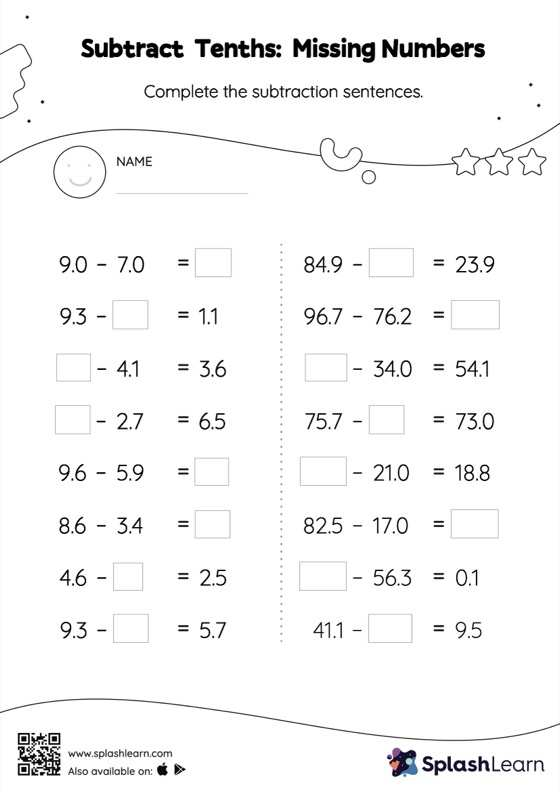# Subtract Tenths: Missing Numbers Worksheet

Home > Subtract Tenths: Missing NumbersWhen subtracting decimals on this worksheet, students align the decimal points and use zero as a placeholder. Then to find the missing number in the subtract tenths worksheet, students then apply the relationship between addition and subtraction. This worksheet is about practicing with the horizontal format in which numbers are written side by side. To develop flexibility with numbers and operations, students need to have enough practice in this format and not just rely on the vertical/column method.